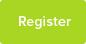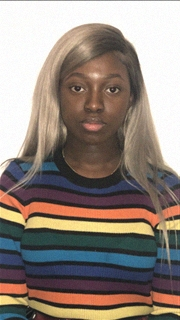# Maths Gcse Questions

Date : 15/03/2019### Author Information

Subject : Maths

Try your hand at these GCSE &questions

1. Which of these shapes has the most sides?

Hexagon

Octagon

Rhombus

Trapezium

Prices:

Pencils - 8p each

Rulers - 30p each

She says,

Then I will buy as many rulers as possible

With my change I will buy more pencils.' How many pencils and how many rulers does she buy?

3. What is 3/2 as a decimal?

1.05

1.1

1.5

3.2

4. An exam has two papers.

Anil scores

33 out of 60 on paper 1

and

75 out of 100 on paper 2

Work out his percentage score for the exam.

5. There are 720 boys and 700 girls in a school.

The probability that a boy chosen at random studies French is 2/3

The probability that a girl chosen at random studies French is 3/5

(a) Work out the number of students in the school who study French.

(b) Work out the probability that a student chosen at random from the whole school does not study French.

6. 3/5 of a number is 162.

Work out the number.

7. Solve the simultaneous equations.

2x + y = 18

x - y = 6

8. PRT and QRS are similar triangles.

(See diagram - not drawn accurately).

(PA)Which of these is equivalent to QR/PR ?

RS/ST

QS/PT

PT/QS

RT/RS

9. To make one cheese sandwich, Gina uses one bread roll and two cheese slices.

- Pack of 15 bread rolls: £1.88

- Pack of 20 cheese slices: £2.15

- She is going to buy enough packs to have exactly twice as many cheese slices as bread rolls.

- Make more than 100 cheese sandwiches.

Work out the least amount she can spend.

10. See graph.

(PA)The graph shows the depth of water in a harbour for 12 hours.

d is the depth of water in the harbour in metres

t is the number of hours after 9 am

(a) For how many of the 12 hours is the depth more than 5 metres?

(b) By how much does the depth change between 12 noon and 4pm?

1. Octagon

2. 17 pencils, 12 rulers

3. 1.5

4. 67.5 or 68

5. (a) 900 (b) 520/1420 or 26/71

6. 270

7. x = 8 and y = 2

8. QS/PT

9. 40.84

10. (a) 8 (b) 3 (or -3)

This resource was uploaded by: Ama